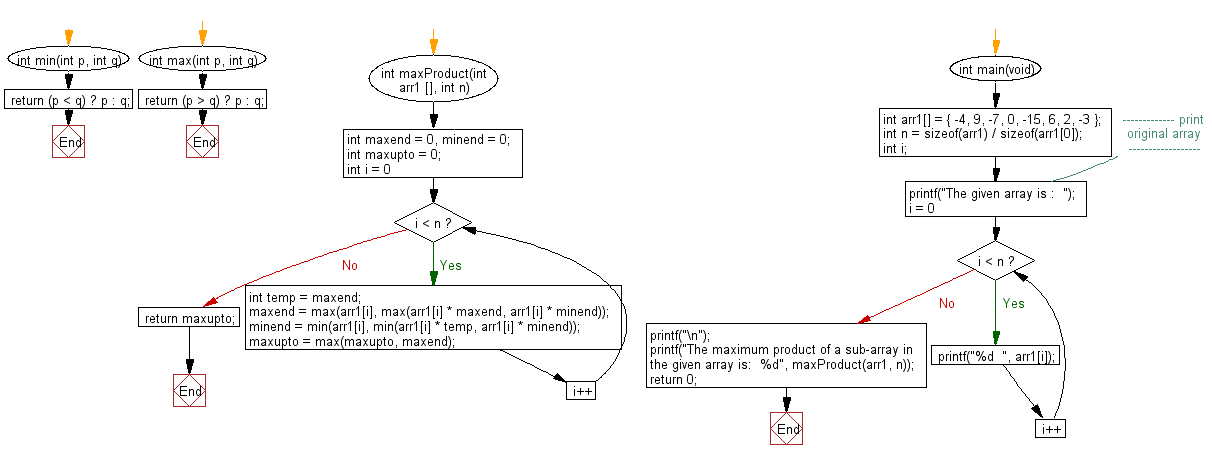﻿ C exercises: Find maximum product subarray in a given array - w3resource# C Exercises: Find maximum product subarray in a given array

## C Array: Exercise-61 with Solution

Write a program in C to find maximum product subarray in a given array.

Sample Solution:

C Code:

``````#include <stdio.h>
int min(int p, int q)
{
return (p < q) ? p : q;
}
int max(int p, int q)
{
return (p > q) ? p : q;
}
int maxProduct(int arr1 [], int n)
{
int maxend = 0, minend = 0;
int maxupto = 0;
for (int i = 0; i < n; i++)
{
int temp = maxend;
maxend = max(arr1[i], max(arr1[i] * maxend, arr1[i] * minend));
minend = min(arr1[i], min(arr1[i] * temp, arr1[i] * minend));
maxupto = max(maxupto, maxend);
}
return maxupto;
}
int main(void)
{
int arr1[] = { -4, 9, -7, 0, -15, 6, 2, -3 };
int n = sizeof(arr1) / sizeof(arr1);
int i;
//------------- print original array ------------------
printf("The given array is :  ");
for(i = 0; i < n; i++)
{
printf("%d  ", arr1[i]);
}
printf("\n");
//------------------------------------------------------
printf("The maximum product of a sub-array in the given array is:  %d", maxProduct(arr1, n));
return 0;
}
```
```

Sample Output:

```The given array is :  -4  9  -7  0  -15  6  2  -3
The maximum product of a sub-array in the given array is:  540
```

Flowchart:C Programming Code Editor:

Improve this sample solution and post your code through Disqus.

What is the difficulty level of this exercise?

﻿